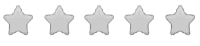See inside this book

# Math-U-See Primer Student Workbook

\$34.00
No tax
Demme Learning
Math
1
P-MA00-62Leave a Review

#### Description

New! Math-U-See Primer - Student Workbook

A gentle introduction to mathematics. Math-U-See is a complete, skill-based, multi-sensory homeschool math curriculum for grades K-12 that?s designed to teach students skills in a logical sequence.

The Primer Student Workbook contains lesson-by-lesson worksheets, systematic review pages, and Application & Enrichment pages.

In the Primer level your child will learn not only how to write numerals but also addition and subtraction, basic counting, skip counting, geometric shapes, telling time, and they will be introduced to the manipulative block system. It?s a gentle introduction to "doing math."

Major Concepts and Skills Include:

Counting objects and developing numeracy Understanding place value Recognizing number names and symbols Reading and writing numerals Understanding addition and subtraction with concrete and representational models Understanding and writing the symbols +, ? and = Addition and subtraction of select numbers

Telling and writing time by hours and minutes Recognizing & drawing rectangles, squares, and circles Measuring length by repeating units Introducing halves and fourths Counting by 2s, 5s, 10s, and 100s Reading, writing & interpreting word problems